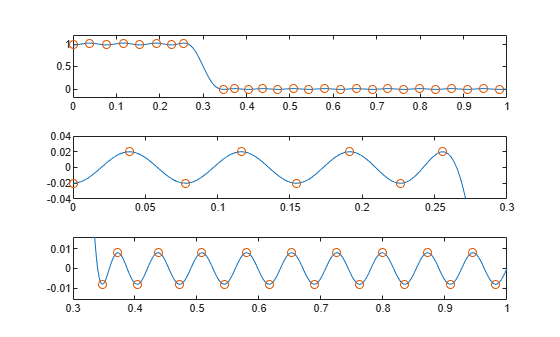# fircls1

Constrained-least-squares linear-phase FIR lowpass and highpass filter design

## Syntax

```b = fircls1(n,wo,dp,ds) b = fircls1(n,wo,dp,ds,'high') b = fircls1(n,wo,dp,ds,wt) b = fircls1(n,wo,dp,ds,wt,'high') b = fircls1(n,wo,dp,ds,wp,ws,k) b = fircls1(n,wo,dp,ds,wp,ws,k,'high') b = fircls1(n,wo,dp,ds,...,'design_flag') ```

## Description

`b = fircls1(n,wo,dp,ds)` generates a lowpass FIR filter `b`, where `n+1` is the filter length, `wo` is the normalized cutoff frequency in the range between 0 and 1 (where 1 corresponds to the Nyquist frequency), `dp` is the maximum passband deviation from 1 (passband ripple), and `ds` is the maximum stopband deviation from 0 (stopband ripple).

`b = fircls1(n,wo,dp,ds,'high')` generates a highpass FIR filter `b`. `fircls1` always uses an even filter order for the highpass configuration. This is because for odd orders, the frequency response at the Nyquist frequency is necessarily 0. If you specify an odd-valued `n`, `fircls1` increments it by 1.

`b = fircls1(n,wo,dp,ds,wt)` and

`b = fircls1(n,wo,dp,ds,wt,'high')` specifies a frequency `wt` above which (for `wt` > `wo`) or below which (for `wt` < `wo`) the filter is guaranteed to meet the given band criterion. This will help you design a filter that meets a passband or stopband edge requirement. There are four cases:

• Lowpass:

• `0` < `wt` < `wo` < `1`: the amplitude of the filter is within `dp` of 1 over the frequency range `0` < ω < `wt`.

• `0` < `wo` < `wt` < `1`: the amplitude of the filter is within `ds` of 0 over the frequency range `wt` < ω < `1`.

• Highpass:

• `0` < `wt` < `wo` < `1`: the amplitude of the filter is within `ds` of 0 over the frequency range `0` < ω < `wt`.

• `0` < `wo` < `wt` < `1`: the amplitude of the filter is within `dp` of 1 over the frequency range `wt` < ω < `1`.

`b = fircls1(n,wo,dp,ds,wp,ws,k)` generates a lowpass FIR filter `b` with a weighted function, where `n+1` is the filter length, `wo` is the normalized cutoff frequency, `dp` is the maximum passband deviation from 1 (passband ripple), and `ds` is the maximum stopband deviation from 0 (stopband ripple). `wp` is the passband edge of the L2 weight function and `ws` is the stopband edge of the L2 weight function, where `wp` < `wo` < `ws`. `k` is the ratio (passband L2 error)/(stopband L2 error)

`$k=\frac{{\int }_{0}^{{w}_{p}}{|A\left(\omega \right)-D\left(\omega \right)|}^{2}d\omega }{{\int }_{{w}_{z}}^{\pi }{|A\left(\omega \right)-D\left(\omega \right)|}^{2}d\omega }$`

`b = fircls1(n,wo,dp,ds,wp,ws,k,'high')` generates a highpass FIR filter `b` with a weighted function, where `ws` < `wo` < `wp`.

`b = fircls1(n,wo,dp,ds,...,'design_flag')` enables you to monitor the filter design, where `'``design_flag``'` can be

• `'trace'`, for a textual display of the design table used in the design

• `'plots'`, for plots of the filter's magnitude, group delay, and zeros and poles. All plots are updated at each iteration step. The O's on the plot are the estimated extremals of the new iteration and the X's are the estimated extremals of the previous iteration, where the extremals are the peaks (maximum and minimum) of the filter ripples. Only ripples that have a corresponding O and X are made equal.

• `'both'`, for both the textual display and plots

Note

In the design of very narrow band filters with small `dp` and `ds`, there may not exist a filter of the given length that meets the specifications.

## Examples

collapse all

Design an order 55 lowpass filter with normalized cutoff frequency 0.3. Specify a passband ripple of 0.02 and a stopband ripple of 0.008. Display plots of the bands.

```n = 55; wo = 0.3; dp = 0.02; ds = 0.008; b = fircls1(n,wo,dp,ds,'both');```
``` Bound Violation = 0.0870385343920 Bound Violation = 0.0149343456540 Bound Violation = 0.0056513587932 Bound Violation = 0.0001056264205 Bound Violation = 0.0000967624352 Bound Violation = 0.0000000226538 `````` Bound Violation = 0.0000000000038 ```

The Bound Violations denote the iterations of the procedure as the design converges. Display the magnitude response of the filter.

`fvtool(b)`## Algorithms

`fircls1` uses an iterative least-squares algorithm to obtain an equiripple response. The algorithm is a multiple exchange algorithm that uses Lagrange multipliers and Kuhn-Tucker conditions on each iteration.

## References

 Selesnick, I. W., M. Lang, and C. S. Burrus. “Constrained Least Square Design of FIR Filters without Specified Transition Bands.” Proceedings of the 1995 International Conference on Acoustics, Speech, and Signal Processing. Vol. 2, 1995, pp. 1260–1263.

 Selesnick, I. W., M. Lang, and C. S. Burrus. “Constrained Least Square Design of FIR Filters without Specified Transition Bands.” IEEE® Transactions on Signal Processing. Vol. 44, Number 8, 1996, pp. 1879–1892.

## Version History

Introduced before R2006a